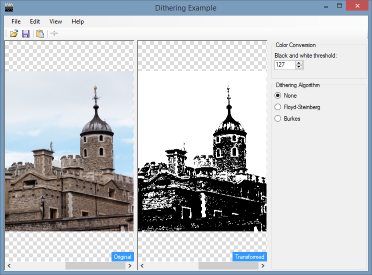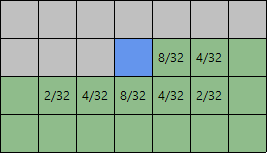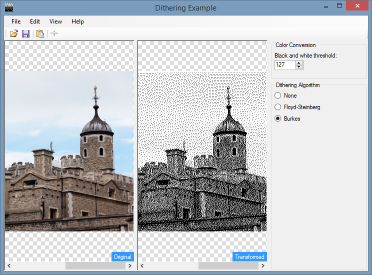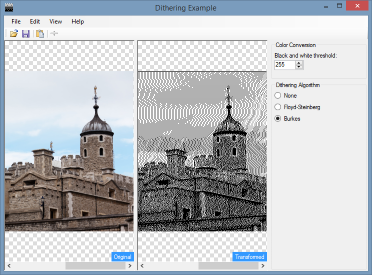## Dithering an image using the Burkes algorithm in C#

In my previous post, I described how to dither an image in C# using the Floyd‑Steinberg algorithm. Continuing this theme, this post will cover the Burkes algorithm.I will be using the same demonstration application as from the previous post, so I won't go over how this works again.

### Burkes dithering

As with Floyd‑Steinberg, the Burkes algorithm is an error diffusion algorithm, which is to say for each pixel an "error" is generated and then distributed to pixels around the source. Unlike Floyd‑Steinberg however (which modifies 4 surrounding pixels), Burkes modifies 7 pixels.

Burkes is actually a modified version of the Stucki algorithm, which in turn is an evolution of the Jarvis algorithms.

The diagram below shows the distribution of the error coefficients.• 8 for the pixel to the right of the current pixel
• 4 for the second pixel to the right
• 2 for the pixel below and two to the left
• 4 for the pixel below and to the left
• 8 for the pixel below
• 4 for the pixel below and to the right
• 2 for the pixel below and two to the right

Unlike Floyd‑Steinberg, the error result in this algorithm is divided by 32. But as that's still a power of two, once again we can use bit shifting to perform the division.

Due to the additional calculations I would assume that this algorithm will be slightly slower than Floyd‑Steinberg, but as of yet I haven't ran any form of benchmarks to test this.

### Applying the algorithm

In my Floyd‑Steinberg example, I replicated the calculations four times for each pixel. As there are now seven sets of calculations with Burkes, I decided to store the coefficients in a 2D array mimicing the diagram above, then iterating this. I'm not entirely convinced this is the best approach, but it does seem to be working.

``````private static readonly byte[,] _matrix =
{
{
0, 0, 0, 8, 4
},
{
2, 4, 8, 4, 2
}
};

private const int _matrixHeight = 2;

private const int _matrixStartX = 2;

private const int _matrixWidth = 5;``````

This sets up the matrix as a static that is only created once. I've also added some constants to control the offsets as I can't create an array with a non-zero lower bound. This does smell a bit so I'll be revisiting this!

Below is the code to dither a single pixel. Remember that the demonstration program uses a 1D array of `ArgbColor` structs to make it easy to read and understand, but you could equally use direct pointer manipulation on a bitmap's bits, with lots of extra code to handle different colour depths.

``````int redError;
int blueError;
int greenError;

redError = originalPixel.R - transformedPixel.R;
blueError = originalPixel.G - transformedPixel.G;
greenError = originalPixel.B - transformedPixel.B;

for (int row = 0; row < _matrixHeight; row++)
{
int offsetY;

offsetY = y + row;

for (int col = 0; col < _matrixWidth; col++)
{
int coefficient;
int offsetX;

coefficient = _matrix[row, col];
offsetX = x + (col - _matrixStartX);

if (coefficient != 0 && offsetX > 0 && offsetX < width && offsetY > 0 && offsetY < height)
{
ArgbColor offsetPixel;
int offsetIndex;

offsetIndex = offsetY * width + offsetX;
offsetPixel = original[offsetIndex];
offsetPixel.R = (offsetPixel.R + ((redError * coefficient) >> 5)).ToByte();
offsetPixel.G = (offsetPixel.G + ((greenError * coefficient) >> 5)).ToByte();
offsetPixel.B = (offsetPixel.B + ((blueError * coefficient) >> 5)).ToByte();
original[offsetIndex] = offsetPixel;
}
}
}``````

Due to the loop this code is now shorter than the Floyd‑Steinberg version. It's also less readable due the coefficients being stored in a 2D matrix. Of course, the algorithm is fixed and won't change so perhaps that's not an issue, but if performance really was a concern you can unroll the loop and duplicate all that code. I'll stick with the loop!

### Final Output

The image below shows our sample image dithered using the Burkes algorithm. It's very similar to the output created via Floyd–Steinberg, albeit darker.Again, by changing the threshold at which colours are converted to black or white, we can affect the output of the dithering even if the conversion is to solid black.### Source Code

The latest source code for this demonstration (which will be extended over time to include additional algorithms) can be found at our GitHib page.

The source code from the time this article was created is available from the link below, however may not be fully up to date.

## Related articles you may be interested in

Filename Description Version Release Date
DitheringTest.zip
• md5: 960d00ff8054b8711bf56c562a141126

Sample C# project demonstrating the Floyd‑Steinberg and Burkes algorithms for dithering an image.

1.0.0.0 06/06/2015